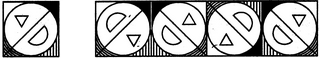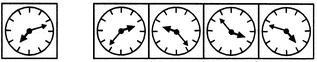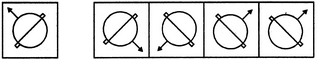# Non Verbal Reasoning - Mirror Images

### Exercise :: Mirror Images - Section 2

In each of the following questions, choose the correct mirror images of the given image of the Fig.(X) from amongst the four alternatives (1), (2), (3) and (4) given along with it.

1.

Choose the correct mirror image of the given figure (X) from amongst the four alternatives.(X)                 (1)          (2)         (3)         (4)

 A. 1 B. 2 C. 3 D. 4

Explanation:

No answer description available for this question. Let us discuss.

2.

Choose the correct mirror image of the given figure (X) from amongst the four alternatives.(X)                 (1)          (2)         (3)         (4)

 A. 1 B. 2 C. 3 D. 4

Explanation:

No answer description available for this question. Let us discuss.

3.

Choose the correct mirror image of the given figure (X) from amongst the four alternatives.(X)                 (1)          (2)         (3)         (4)

 A. 1 B. 2 C. 3 D. 4

Explanation:

No answer description available for this question. Let us discuss.

4.

Choose the correct mirror image of the given figure (X) from amongst the four alternatives.(X)                 (1)          (2)         (3)         (4)

 A. 1 B. 2 C. 3 D. 4

Explanation:

No answer description available for this question. Let us discuss.

5.

Choose the correct mirror image of the given figure (X) from amongst the four alternatives.(X)                 (1)          (2)         (3)         (4)

 A. 1 B. 2 C. 3 D. 4